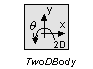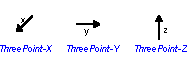﻿ 20-sim webhelp > Library > Iconic Diagrams > Mechanical > Translation > 3DSmallRotation > ThreeDLibrary

# ThreeDLibrary

Navigation:  Library > Iconic Diagrams > Mechanical > Translation > 3DSmallRotation >

# ThreeDLibrary## Library

The 3DSmallRotation library contains 3D models that are only suited for small rotations. The library is comparable to the 2DSmallRotation library and suited for modeling systems that, due to limited stiffness, experience rotation that should be accounted for.

## Degrees of Freedom

All models in 3DSmallRotation library contain one or more ports with 6 degrees of freedom. The first three degrees of freedom denote the x-, y- and z-position and the last three degrees of freedom denote the rotation around the x-, y- and z-axes. A vector notation is used to denote the forces and velocities for these degrees of freedom. For a model with a port P the notation is:

 degree identity forces velocities x position P.F [N] P.v [m/s] y position P.F [N] P.v [m/s] z position P.F [N] P.v [m/s] qx angle P.F [Nm] P.v [rad/s] qy angle P.F [Nm] P.v [rad/s] qz angle P.F [Nm] P.v [rad/s]

### Note

 • It is not possible in 20-sim to use vector elements with mixed units. Therefore element number 4 to 6 will be displayed with units [m/s] and [N] although it really is [rad/s] and [Nm]!
 • The vector element number (1 to 6) denotes the identity of the degree of freedom. Flipping or rotating of a model does not change the this identity (e.g. a rotation does not change x into y). Preferably leave the orientation of the models as they pop up on the screen.

## 3D-bodyThe central model in the 3DSmallRotation library is the 3D-body model which describes a body with 6 degrees of freedom.

## 3D-mass

The 3D-mass model is a body with only mass and zero rotational inertia.

## PointsThe 3D-body model is nothing more than a center of mass. To connect a 3D-body with the outside (single degree of freedom) world, 3D-points are used. A 3D-point describes the offset between the center of mass and the connection point.

 • A 3D-body has to be connected to 3D-points with at least three orthogonal nonzero offsets to prevent it from free rotation.
 • A 3D-body has to be connected to at least three orthogonal 3D-point models to prevent it from free motion.

## Sensors

The velocities of bodies can be inspected directly in the body models. To find positions sensor models are available.